Courses

# Raoult’s Law for VLE Civil Engineering (CE) Notes | EduRev

## Civil Engineering (CE) : Raoult’s Law for VLE Civil Engineering (CE) Notes | EduRev

The document Raoult’s Law for VLE Civil Engineering (CE) Notes | EduRev is a part of the Civil Engineering (CE) Course Thermodynamics.
All you need of Civil Engineering (CE) at this link: Civil Engineering (CE)

Raoult’s Law for VLE

As mentioned in the concluding part of the last section, vapour-liquid systems which are ideal in nature display a behaviour corresponding to Raoult’s Law. In such a system, both the vapour and the liquid phases essentially behave as ideal mixtures. For describing the VLE for such systems we start by applying eqn. 6.50, to the vapour and liquid phases: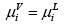...(7.20)
For an ideal vapor mixture by eqn. 6.72 we have: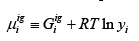Similarly for an ideal liquid solution eqn. 6.77 provides: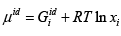Thus rewriting eqn. 7.20 (using expressions provided by eqns. 6.72 and 6.77):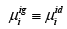...(7.21)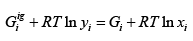...(7.22)

Or :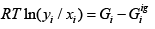...(7.23)

Since effect of pressure is negligible on liquid properties we assume that: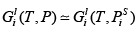...(7.24)

Now for the gas phase: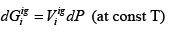...(7.25)

Thus: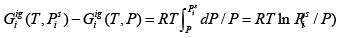...(7.26)

Combining eqns.7.23, 7.24 and 7.26 gives: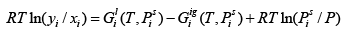...(7.27)

The first two terms on the RHS in equation above correspond to the Gibbs free energy of pure liquid and vapour phases under equilibrium conditions, i.e., at (T,Pi s ) ; hence, as shown in section 7.1, these terms equal. Therefore, it follows that: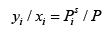...(7.28)

Alternately: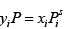...(7.29)

Equation 7.29 is known as the Raoult’s Law.

It may be noted that the conditions for ideal mixture behaviour for the gas and liquid phases are not the same in general. For the gas mixture to be ideal the pressures need to be close to atmospheric or less. While a liquid solution is ideal if the interaction between the same molecular species is identical to that between dissimilar molecules.

The algorithms needed for generating RL phase diagrams are discussed later in this section. But prior to that, we present examples of typical phase diagrams that obtain from the application of the Raoult’s law (RL) equations. Consider again a binary system for which a representative isothermal plot is depicted in fig. 7.7. (The more volatile of the two components is designated as component ‘1’). The upper straight line represents the saturated liquid compositions, while the lower curve corresponds to the saturated vapour compositions. At any pressure, the phase compositions are found at the intersections of a line parallel to the x-y axis with the P-x and P-y curves. The straight line connecting these compositions is the tie line (such as A1-B1, A2-B2, etc.). The portion of the diagram enclosed by the P-x-y curves corresponds to the two phase region where the vapour and liquid phases co-exist. Any point lying outside of this two-phase envelope corresponds to a state where only a single phase is present. Now consider the point L which lies above the phase envelope. At this condition the mixture exists as a compressed (or sub-cooled) state whose composition corresponds to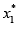. Lowering the pressure at this fixed composition eventually brings the liquid mixture to the point A3, where any further reduction of pressure leads to the formation of a vapour phase whose composition is given by B3. Thus this point is characterized by the formation of the first bubble of vapour, and hence is termed the bubble point, the corresponding pressure being the bubble pressure, at the given composition.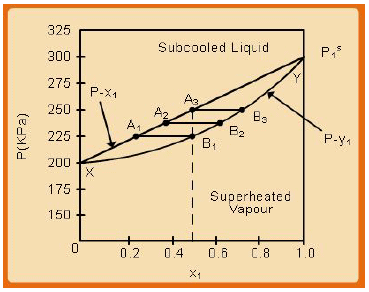Fig. 7.7 Model P-x-y plot for a system obeying Raoult’s Law

Next consider the point V in the above diagram. At this state the mixture is at the same overall composition as at L, but the state is one of single phase, superheated vapour. Increasing the pressure at the same composition eventually brings the mixture to the point B1, where any further increase of pressure leads to the formation of the liquid phase, whose composition is given by the point A1 *Thus this point is said to be the dew point corresponding to the vapour phase composition given by while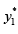the pressure at this point is termed the dew pressure.

We revert to the discussion on the system state at the bubble point A3 . If one reduces the pressure progressively formation of more bubbles of vapour occurs, which coalesce and lead to the development of a bulk vapour phase.  The system eventually reaches the point B1 where practically the entire mixture exists in the vapour form; further reduction of pressure renders the mixture superheated and finally one reaches the point V (and beyond). Let us focus on what happens as the system transits through the two-phase region defined by the end points A3 and B1 . Note that the overall composition of the system remains invariant as the pressure reduces. However, since now the original amounts of each species need to be distributed across the co-existing vapour and liquid phases the actual composition in each phase must change in accordance with the following mass conservation equation: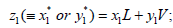; where, z1 = overall composition; x1 and y1 are compositions of the liquid and vapour phases at equilibrium, and L andV are the relative amounts of moles (per mole of the original mixture) in the liquid and vapour phases, respectively (thus L +V= 1) . The liquid phase composition progressively changes along the line A3 to A1 (bubble curve), while the composition of the vapour phase in equilibrium with the liquid phase transits from B3 to B1 (dew curve). At each pressure between the bubble pressure (Pb) and the dew pressure (Pd) the equilibrium vapour and liquid phase compositions, as well as the relative amounts of mass in each phase are constrained by the relations: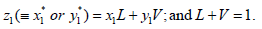The associated, isobaric T-x-y plots for the same system are shown in fig.7.7. As is expected the dew temperature curve lies above the bubble temperature curve. The lens-like region corresponds to the two-phase states of the system. At the point V the system is in a single phase, super-heated state.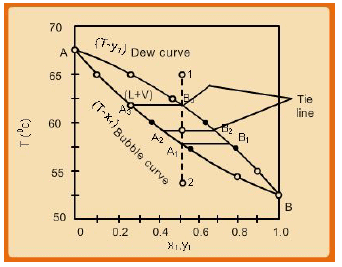Fig. 7.8 Model T-x-y plot for a system obeying Raoult’s Law

Progressive reduction of temperature brings it to the point B 3 where the first dew of liquid forms, whose composition is provided by the point A3. Thus this point is referred to as the dew point, and the corresponding temperature called the dew temperature(Td ) for the given vapour phase composition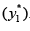.On the other hand if one starts from the point L (single-phase, compressed liquid state) gradual increase of temperature brings the system to A1, the bubble point, where the first bubble of vapour forms. The associated temperature then is the bubble temperature (Tb) for the composition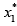.At any other temperature intermediate to Tand Tb, the system contains co-existing vapour and liquid phases whose compositions are constrained by the same mass conservation relations provided above, i.e.,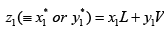and L +V= 1. Note that as in the P-x-y plot the T-x-y plots also are characterized by horizontal tie lines that connect the compositions of the equilibrated vapour and liquid phases.

The data in figures 7.7 and 7.8 may be may be alternately displayed in the form of a y-x plot (fig. 7.9). It shows the equilibrium vapour and liquid phase compositions in a more immediate manner. Note that, as required by the phase rule, each pair of equilibrium y and x values correspond to a different combination of equilibrium temperature and pressure.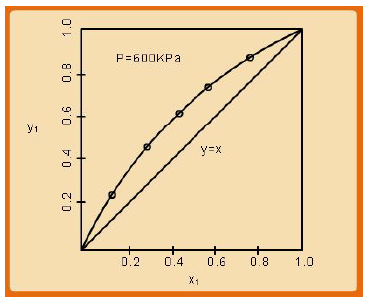Fig. 7.9 Model y-x plot for a system obeying Raoult’s Law

Multi-component VLE calculations using Raoult’s Law: For generality we consider a system containing N chemical species. Then by phase rule, for a VLE situation, the degrees of freedom are 2N, the phase rule variables being {xi},{yi}, T, and P. The Raoult’s Law (eqn. 7.29) provides N constraining relations connecting these variables. Thus, for solving the VLE problem, N variables need to be specified, so that the values of the other N variables may be determined. Five types of VLE computations are commonly encountered in practice. They are enumerated below in table 7.1 first and then the relevant algorithms used are presented.

Table 7.1 Types of VLE calculations

 VLE Type Specified Variables Computed Variables Bubble Pressure T and {xi} P and {yi} Dew Pressure T and {yi} P and {xi} Bubble Temperature P and {xi} T and {yi} Dew Temperature P and {yi} T and {xi} Flash Distillation T,P and {zi} L or V, {xi} and {yi}

In the above table the notations used signify the following:

{ xi } ≡ overall liquid phase compoition { xi , x2  , xN-1 }
{ yi } ≡ overall liquid phase compoition { yi , y2  , yN-1 }
{ zi } ≡ overall feed composition to flash vessel { zi ,z2 ,  zN-1 }
L ≡ moles of liquid phase formed per mole of feed to flash vessel

In summary, therefore, one specifies either T or P and either the liquid-phase or the vapor-phase composition, thus fixing 1+(N-1) or N phase rule variables as required by the phase rule for VLE calculation. The variants of Raoult’s Law (eqn. 7.29) which are used are as follows: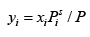......(7.30)

Thus: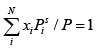Or: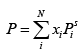......(7.31)
Also: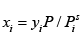......(7.32)

Thus: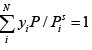Or: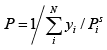......(7.33)
For computation of vapour pressures the Antoine equation (or another suitable equation) may be used: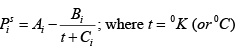......(7.34)

Bubble Pressure: Given T and {xi} , to calculate P and {yi} :

a) Use eqn. 7.31 to compute P

b) Next use eqn. 7.30 to obtain {yi}

Dew Pressure: Given T and {yi} , to calculate P and {xi} :

a) Use eqn. 7.33 to compute P

b) Use eqn. 7.32 to obtain {xi}

Bubble Temperature: Given P and {xi} , to calculate T and {yi}

a) For the given pressure compute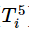using the following form of Antoine eqn.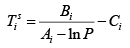b) Initialize the bubble temperature as: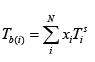c) Using computed T calculate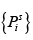d) Use equation 7.30 to compute { yi }

e)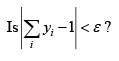= pre - defined acceptable error for convergence)

f)  If yes,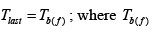= final acceptable bubble temperature

g) At Tb(f)  compute final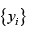h) If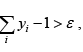then the assumed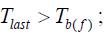revise to new T as: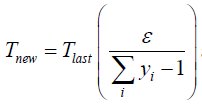and return to step (c).

i) if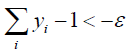, the the assumed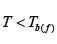;  where Tb(f) = final acceptable bubble temperature

Revise to new T using: Tnew =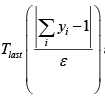and return to step (c).

Dew Temperature:

Given P and {yi} , to calculate T and {x}

a) For the given pressure compute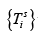using the following form of Antoine eqn.

b)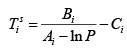c) Initialize the dew temperature as: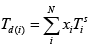d) Using computed T calculate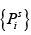e) Use equation 7.33 to compute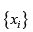f)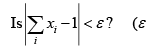pre - defined acceptable error for convergence)

g) If yes,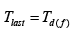; where Tb(f) = final acceptable bubble temperature

h) At Tb(f) ) compute finalj) If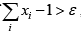then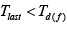; revise to new T as: :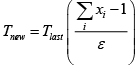and return to step (c).

k) If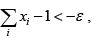then; revise to new T as: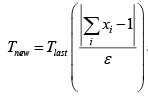and return to step  (c).

Flash Distillation Calculations : This is an operation, often exploited in the chemical industry to achieve the desired enrichment of a feedstock through a one-step distillation process. A schematic of the process is shown in fig. 7.6. A liquid at a pressure equal to or greater than its bubble point pressure is introduced into the flash by passing it through a pressure reduction valve. The abrupt reduction in pressure "flashes" or partially evaporates the feed liquid, which results in the formation of a vapour and a liquid stream which are typically assumed to leave the flash vessel in equilibrium each other.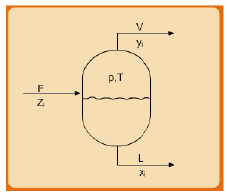Fig. 7.10 Schematic of Flash Distillation Process

One of the common forms of flash calculation typically involves the determination of the liquid and vapour stream composition that results from process as well also the resultant liquid or vapour phase mole fractions that obtains per mole of feed. Consider a system containing one mole of mixture of chemical species with an overall composition represented by the set of mole fractions { zi } . Let L and V be the moles of liquid and vapour formed per mole of feed. The corresponding stream compositions are denoted as { xi} and { yi} respectively. The material-balance equations are:

F = L +V= 1                              ........(7.35)
zi F = xi L + yiV (1 )
zi = xi − V+ yiV                            ........(7.36)

Now: Ki = yi / xi =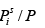........(7.37)

Putting xi = yi /Ki and using relations in (7.44) one obtains: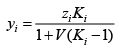........(7.38)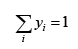Using this condition in eqn. 7.38: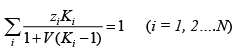........(7.39)

Since xi = yi /Ki , an alternative equation is: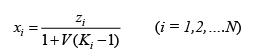........(7.40)

It follows that: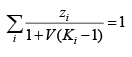........(7.41)

Subtracting eqn. 7.41 from 7.39and defining a functionϕ we get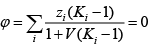........(7.42)

It follows that: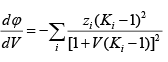........(7.43)

The derivative d dV ϕ is always negative; in other words the relation between ϕ vs. V is monotonic, and this makes for convenient application of the well-known Newton-Raphson method of solution  this leads to the following equation for the  nth teration: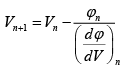........(7.44)

Where, the values for ϕ and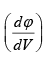may be computed using eqns. 7.42 and 7.43 respectively.

Offer running on EduRev: Apply code STAYHOME200 to get INR 200 off on our premium plan EduRev Infinity!

## Thermodynamics

56 videos|92 docs|33 tests

,

,

,

,

,

,

,

,

,

,

,

,

,

,

,

,

,

,

,

,

,

;